International Journal of Geomagnetism and Aeronomy
Vol 1, No. 3, August 1999

# On the problem of quasi-resonant wave trapping in the LHR waveguide

F. Jiricek

Institute of Atmospheric Physics, Academy Science of the Czech Republic, Prague, Czech Republic

D. R. Shklyar

Institute of Terrestrial Magnetism, Ionosphere, and Radio Wave Propagation, Troitsk, Moscow Region, Russia

### Abstract

A theory of lower hybrid resonance (LHR) waveguide feeding by quasi-resonant VLF waves is developed. The wave trapping in the waveguide is studied in the framework of two-dimensional geometrical optics. Properties of LHR frequency profiles necessary for wave trapping in the LHR waveguide are elucidated. Analytical consideration permits understanding some specific outcomes of ray tracing, which uses typical LHR frequency profiles. Theoretical results are illustrated by an example of satellite registration of the VLF band, where both the wave electric field and plasma parameters are available from Intercosmos 24 data.

### Introduction

Lower hybrid resonance (LHR) waves [Brice and Smith, 1965] are commonly found in VLF wave data obtained from satellites in the outer ionosphere and the magnetosphere (e.g., Laaspere and Taylor, 1970]). LHR noise observed in early satellite experiments was interpreted by Smith et al. to result from the trapping of whistler energy in the valley of LHR frequency altitude profile [Kimura,1985], or in the ionospheric duct, as it was originally called [Smith et al.,1966]. Later in this paper, we use the term LHR waveguide for the corresponding profile of LHR frequency.

Later on, other mechanisms of LHR wave generation were suggested, namely, wave excitation by energetic electron streams (e.g., [Horita and Watanabe,1969; Horita,1972]) and linear and nonlinear VLF wave scattering on small-scale plasma turbulence (see, for instance, Bell and Ngo[1990, and references therein] and Trakhtengerts and Hayakawa, and references therein). We will not further discuss these processes here, since in this paper we deal only with the above mentioned mechanism suggested by Smith et al.. Since these ideas had been put forward, the knowledge of LHR waveguide has been widely used in interpreting VLF data from satellite measurements (e.g., [Jirícek and Tríska,1976; Nishino and Tanaka,1987]).Although wave propagation inside the waveguide was considered in many papers beginning with the pioneering work by Smith et al., the mechanism of the waveguide feeding remained unclear. That question is dealt with in this paper. To formulate the problem in more detail, we recall the well-known features of quasi-resonant LHR waves [Stix, 1962]: large (close to p/2 ) wave normal angle q with respect to an ambient magnetic field B0 ; short wavelength corresponding to large refractive index (more precisely, k2 c2/wp2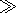1, where k is the wave number, wp is electron plasma frequency, and c is the speed of light); and the quasi-electrostatic character of the wave field. Such a wave cannot propagate in the region where its frequency w is essentially smaller than the local LHR frequency wLH (see dispersion equation (5) and relation (7) below). A typical LHR frequency profile in the outer ionosphere is shown in Figure 1. According to the tradition of representing this profile, the altitude is along the vertical axis, while the frequency wLH is along the horizontal one. In the plane ( wLH, altitude), the wave trajectory corresponds to a vertical line w = const. If the wave moves towards increasing values of wLH, its group velocity decreases, and the wave is reflected in the vicinity of the point where this line crosses the LHR frequency profile. Thus waves with frequencies (wLH)min < w< (wLH)max, if they exist in the waveguide (that is, below hmax ), would be trapped inside the waveguide. But, at first sight, the waveguide could not be fed either from above or from below, and the quasi-resonant LHR waves described above should be excited directly inside the waveguide. As was mentioned above, the corresponding mechanisms of local excitation of LHR waves were suggested, namely, scattering of upgoing waves on small-scale turbulence or plasma stream instability. However, these local mechanisms require some additional conditions to be fulfilled in the waveguide region, namely, the existence of small-scale plasma turbulence or density irregularities in the case of wave scattering or the existence of energetic electron streams in the case of instability. At the same time, the most natural way of waveguide feeding would be wave penetration from higher altitudes, where quasi-resonant VLF waves propagating in a nonducted mode normally exist. In this paper we suggest a mechanism of LHR waveguide feeding by those waves and give evidence for this mechanism derived from ray tracing calculations and satellite observations. The analysis is based on the solution of the equations of geometrical optics, with the account of a realistic two-dimensional shape of the LHR frequency profile. We should emphasize underline that we consider wave propagation only in the upper ionosphere and the magnetosphere, while wave propagation in the lower ionosphere, where the LHR frequency essentially decreases owing to exhaustion of the ionized plasma component, is beyond the scope of the present work. Accordingly, in Figure 1 and Figure 4 below, we do not show LHR profiles in this region of the lower ionosphere (heights h 100-200 km).

### 2. Quasi-Resonant Whistler Mode Wave Propagation in the LHR Frequency Band

We remind the reader that the LHR frequency in the outer ionosphere (from, say, 500 km up to a few thousand kilometers) ranges from a few kHz up to above 10 kHz, and usually it has the characteristic shape of its profile as shown in Figure 1. Thus waves in the LHR frequency range belong to the VLF frequency band (1-30 kHz). As for the mode of propagation in the magnetosphere, these waves correspond to whistler mode waves, as a few tens of kHz is a characteristic value of the equatorial electron gyrofrequency in the plasmasphere ( L shells from about 2 to 4, where L is McIlwain's parameter). Owing to essential increases of electron gyrofrequency wH with decreasing heights, the same waves fall in the LHR frequency band in the ionosphere.

Using a general equation for the wave refractive index in a cold, magnetized plasma [Stix, 1962], one can find the dispersion relation for whistler mode waves in the frequency range wHi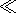wwHwHi is the ion gyrofrequency):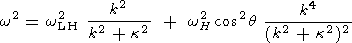(1)

where LHR frequency wLH is determined by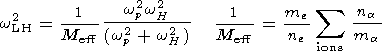(2)

ne and me are electron concentration and mass, respectively, and na and ma are the same for ions of species a ), and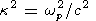(3)

From (1) one can see that the characteristic value of the wave number for whistler mode waves is k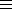wp/c. For the so-called quasi-longitudinal waves [Ratcliffe,1959; Helliwell,1965] k k, while the inequality kk corresponds to quasi-resonant waves (see, for example, Shklyar and Washimi ). In general, whistler mode waves exist when w > wLH as well as when w< wLH ; however, the last range is accessible only for waves in the quasi-longitudinal mode of propagation. At the same time, quasi-resonant waves having k2k2 and wave normal angles q close to p/2 are very sensitive to the LHR frequency profile.

To consider the propagation of quasi-resonant waves in the LHR frequency range, we simplify the dispersion equation (1), assuming(4)

which gives(5)

where k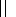and k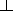are the longitudinal and transversal components of the wave vector, respectively, and(6)

We should mention that the last inequality in (4) is in fact implied by the previous ones. From (5) it is easy to see that for the waves under discussion(7)

where (4) and (2) have been taken into account. Equation (5) constitutes the basis for analysis of the quasi-resonant wave propagation in the LHR frequency band.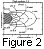We will restrict ourselves to consideration of wave propagation in the meridional plane and will suppose the Earth's magnetic field to be dipolar. As is well known (e.g., [Fermi,1968]), the equations of geometrical optics can be written in Hamiltonian form with the components of coordinates r and wave vector k being canonically conjugate variables. The Hamiltonian from which these equations should be derived is the wave frequency w expressed through the variables r, k according to the dispersion relation (in our case, equation (5)). Since the quantities kand kwhich enter (5) are expressed through Cartesian coordinates and wave vector components in a rather complicated way, the Cartesian variables are not the proper ones for our problem. That is why we will use dipolar coordinates L, M, connected with Cartesian coordinates (x, z) by the relations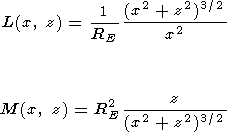(8)

Here RE is the Earth's radius; the origin of the Cartesian coordinates is supposed to be at the Earth's center, the z axis pointing along the dipolar axis from S to N. The coordinate L is McIlwain's parameter, which is constant along the field line; the coordinate M is measured along the field line from the equator (see Figure 2). The Lamé coefficients corresponding to the coordinates L, M are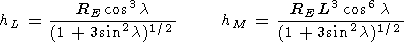(9)

where l is the geomagnetic latitude: tan l = z/x.

The momenta PL, PM canonically conjugate to the coordinates L, M are related to the transverse and longitudinal wave vector components k, kby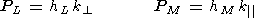(10)

In new variables (L, M, PL, PM), the expression for the wave frequency (5) playing the role of the Hamiltonian from which the equations of geometrical optics can be derived takes the form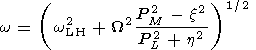(11)

where the following notation has been introduced(12)

and it is supposed that the quantities wLH, W, x, and h are expressed as the functions of coordinates L, M. Using (4) and notation (12) and taking into account (wLH2 /wH2)1, we obtain the relations between different quantities entering (11):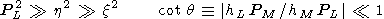(13)

We now write the equations of geometrical optics which follow from (11):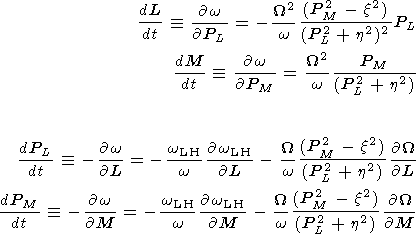(14)

While deriving equations (14) for momenta PL and PM, we have made use of the fact that, under conditions (13), the derivatives of the quantities x2 and h2 can be neglected.

The physical meaning of the derivatives dL/dt and dM/dt is revealed by the relations which connect these quantities with transversal and longitudinal components of the group velocity. Since hL dL is equal to the length across the geomagnetic field line corresponding to the variation dL of the coordinate L at constant M and hM dM is the length along the field line corresponding to the variation dM at constant L, then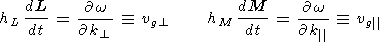(15)

which, of course, is equivalent to the first two equations in (14) in view of the relations (10).

We proceed with the analysis of the wave propagation near the LHR frequency by comparing the longitudinal and transversal group velocities (15). From (14) it follows that the ratio between these two quantities depends on the magnitude of the longitudinal momentum PM. We will relate the value of PM2 to the quantity x2, keeping in mind that in view of limitations (13) the relation between PM2 and x2 may be arbitrary. We divide the range of the permitted values of the quantity PM2 into two domains: PM2 x2 and PM2 < x2. From (13) and (14) it is easy to see that for PM2 x2, |hMdM/dt||hLdL/dt| ; thus the wave group velocity is almost aligned with the ambient magnetic field. For PM2 < x2, | vg| is still larger than | vg| unless PM2 is as small ashL2 x4/hM2 PL2, and for smaller PM2, | vg| > | vg|. Thus on the greater part of the wave trajectory, | vg| is larger than |vg|, which permits, in some cases, the reduction of the problem to a one-dimensional one. This has been done in the papers by Bosková et al. [1988, 1992]. On this basis, the explanation of the lower hybrid resonance whistler traces has been attacked. However, for the problem discussed here, such an approach is not pertinent. Thus in this study, we will abandon the limitation of one-dimensional wave propagation.

It follows from (14) that the sign of vgdepends on the sign of PL ; namely, they have the opposite signs for (PM2 - x2) > 0 and the same signs for (PM2 - x2) < 0. As was shown by ray tracing calculations [Walter and Angerami, 1969], the wave normal vector of the nonducted whistler wave turns antiearthward while propagating in the magnetosphere (for realistic models of plasma distribution). Thus the wave reaches the outer ionosphere after propagating from one hemisphere to another with PL > 0 (see (10)). For these waves, which we consider later on, vg< 0 on the greater part of a trajectory, so that the wave moves predominantly toward lower L shells. As for vg, it has the same sign as PM, and both change sign at the wave reflection point (over the longitudinal coordinate M ).

### 3. The Mechanism of Wave Trapping in the Waveguide

No matter that the wave group velocity is almost aligned with the ambient magnetic field, even slow motion of the wave across the field lines is essential to our problem. Thus we shall consider a two-dimensional problem, although the dependence of the quantities upon the coordinate L may be considered as a slow dependence on the time scale of the wave longitudinal motion.

We now rewrite the equations (14) in the form(16)

where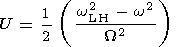(17)

The qualitative physical mechanism of the wave trapping into the waveguide can be understood from the well-known analogy between geometrical optics and mechanics [Fermi,1968], where mass point corresponds to the wave packet, and particle trajectory corresponds to the ray path. Equations (16) for M and PM resemble the equations of particle motion in the potentional of the kind (17) which has a maximum and a minimum over the M coordinate, corresponding to the maximum and minimum of the LHR frequency profile, and a potential wall, corresponding to the bottom part of the profile (see Figure 1). Since the problem is two dimensional, the effective potential also depends on the coordinate L as a parameter. Once passing near the potential maximum, the particle continues to move over both M and L coordinates. Then if the potential maximum increases, the particle can be trapped after reflection from the potential wall. A monotonic increase of the potential maximum, connected with a monotonic variation of the LHR maximum with L coordinate, guarantees that the particle will stay in the trap and, correspondingly, the wave will remain in the waveguide.

For a precise description of the wave trapping, a more strict consideration is needed. Toward this direction, we proceed in the following way. Since the Hamiltonian (11) is independent of time and, thus, is a constant of motion, the problem can be reduced to a one-dimensional one in a standard way (see, for example, Arnold ). We suppose the quantityU /L to be sign determined and choose PL as a new independent variable. Dividing the equations (14) for M, and PM by (dPL/dt) and excluding the quantity L with the help of the integral (11), we arrive at two equations for the variables M and PM as functions of PL. These equations are derived from the Hamiltonian H = L(M, PM; PL, w), which (up to arbitrary constant) is nothing but the coordinate L expressed from (11) as a function of M, PM; PL, and parameter w. To find the function L(M, PM; PL, w), we rewrite the relation (11) in the form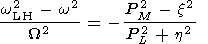(18)

For the sake of simplicity, we neglect the dependence of the quantities x2 and h2 on coordinate L. As was shown above, the variation of coordinate L is small; thus we may expand the expression on the left-hand side in (18) around initial coordinate L0. It is convenient to write this expansion in the form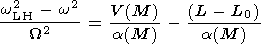(19)

Putting this expansion into (18), we find the quantity (L - L0) which plays the role of the Hamiltonian in the problem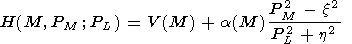(20)

In our case, the role of independent variable is played by the quantity PL, whereas the usual independent variable in the equations of motion is time. To restore the habitual notation, we denote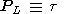(21)

The Hamiltonian (20) depends on t and, thus, varies along the wave trajectory. At the same time, according to the definition of H as L - L0, its initial value at L = L0 is equal to zero.

We proceed with the analysis of the equations derived from (20), keeping in mind that the equations of geometrical optics describe the wave propagation in real space with a group velocity, the role of momentum being played by a wave vector. Positive direction of the M axis in the northern hemisphere is from the equator to the Earth (see Figure 2), so that increasing M values correspond to decreasing heights. We will consider waves having positive PM above the LHR maximum and, thus, propagating to the Earth.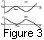To get an idea about the shape of potential V(M), we notice that according to (19)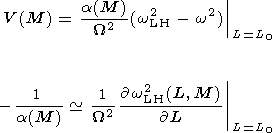(22)

It is natural to assume that a(M)/W2 is a slowly varying (as compared to wLH2 ), sign-determined function. Thus roughly speaking, the shape of potential V(M), up to the sign of a(M), is similar to the shape of the LHR frequency profile. The qualitative shape of the potential V(M) is schematically illustrated in Figure 3a and Figure 3b for a > 0 and a< 0, respectively. We should mention that a > 0 corresponds to an increase of LHR frequency with decreasing L shell, and vice versa.

We can now give a simple, but strict, interpretation of the wave trapping into the wave-guide. The equations for M, PM as functions of the independent variable t which follow from (20) have the form(23)

At the same time, the Hamiltonian (20) itself varies with t according to the equation(24)

Let us first consider the case a > 0, when the potential V(M) has the shape represented in Figure 3a. Using the definitions of the quantities V (equation (22)) and x2 (equation (12)) and inequalities (13), one can easily show that DVV(M)ax2/(t2 + h2), where DV is the variation of potential across the LHR profile. From (20) it then follows that the wave cannot penetrate deeply under the potential wall. At point Mmax, corresponding to the maximum of the potential V(M), let the quantity H (equation (20)) be larger, but close to Vmax. (We remind the reader that initially H = 0, while Vmax(wLH2 - w2)max, so that such a situation appears when w (wLH)max.) In this case the wave passes over the potential maximum. Then the quantity H varies according to (24), and as was shown above, for a > 0 it decreases over the greater part of the profile. Thus the wave becomes trapped between the potential wall and the potential maximum. In this consideration we assumed tPL >0, in agreement with general properties of nonducted whistler wave propagation (see the end of section 2).

Let us turn to the case a< 0. One should now be cautious in using a similarity with particle motion in a potential, since the case a< 0 formally corresponds to the motion of a particle with negative mass. As before, the initial value of the Hamiltonian H (equation (20)) is equal to zero, while the shape of potential V (Figure 3b) is reversed as compared with the case a > 0. Using similar arguments as above, one can show that in the case a< 0, the wave cannot penetrate far above the potential minimum Vmin, while trapped waves correspond to H > Vmin. Since initially H < Vmin, for a wave to be trapped, an increase of the quantity H along the wave trajectory would be necessary. However, it is not the case when a< 0. Indeed, from (16) and (22) it follows that for a< 0, dPL/dtdt/dt < 0, so that the value of t decreases along the wave path. Equation (24) then shows that during the time of wave crossing of the LHR profile, the quantity H decreases on the whole. Thus the wave cannot be trapped in the waveguide in the case a< 0, that is, when the LHR frequency decreases with a decrease of L shell. We should stress that, independently of the sign of a, the quantity PLt is large (as compared with PM and h ) and positive and that, on the average, PM2x2. These facts have been assumed in the analysis of trapping conditions.

To summarize, the possibility for LHR waveguide feeding by nonducted VLF waves propagating in the magnetosphere appears when the two-dimensional LHR frequency profile satisfies the following requirements: the profile of the LHR frequency along a given geomagnetic field line (i.e., the line L = const) has a valley lying between local maximum, local minimum, and the bottom wall, and the value of local maximum along the field line increases with decreasing L shells.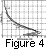Figure 4 shows model LHR frequency profiles which satisfy these requirements. The LHR frequency (horizontal axis) is plotted as a function of height from the Earth (vertical axis) along the geomagnetic field lines L = 2, 3, and 4. Similar to Figure 1, the lower ionospheric heights are not shown in Figure 4.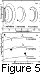Examples of wave trajectories corresponding to wave trapping in the LHR waveguide, obtained from the ray tracing calculations using the profiles of Figure 4, are shown in Figure 5. We should emphasize that they are model ray tracing calculations, since they use analytical LHR profiles shown in Figure 4 that resemble the calculated profile in Figure 1 and they satisfy the above formulated conditions for the wave trapping. Figure 5a shows the ray paths in Cartesian coordinates (x, z) where the trapping is manifested as line broadenings in the near-Earth region; Figure 5b displays the same wave trajectories in (L, M) coordinates where the wave trapping is most evident. When the wave becomes trapped in the LHR waveguide, the longitudinal coordinate M of the wave trajectory starts oscillating, while the transversal coordinate L decreases on the average, in agreement with the analytical results of section 3. We should stress that the quasi-resonant mode of propagation and proper features of LHR profiles do not guarantee wave trapping: the wave may well be reflected either above hmax or even from the bottom of the profile, but still leave the waveguide. For a wave to be trapped, it should have a frequency sufficiently close to the local maximum of the LHR frequency along the wave path. That is why the wave may be trapped after one or several hops in the magnetosphere, when appropriate conditions for trapping arise.

The trajectory marked "1 hop trapping" gives an example of wave trapping in the LHR waveguide after one hop between conjugate hemispheres. The wave propagates in the magnetosphere in a nonducted mode, enters a quasi-resonant regime of propagation, and crosses the LHR maximum in the opposite hemisphere having a frequency slightly higher than (wLH)max. While the wave continues moving toward the bottom of the profile, at the same time it shifts to lower L shells at which the LHR maximum increases. After reflection from the bottom of the profile, the wave approaches the maximum of the LHR profile with w< (wLH)max at which quasi-resonant waves cannot propagate. The wave is reflected from the LHR maximum and, thus, becomes trapped in the waveguide.

The trajectories marked "2 hop trapping" and "3 hop trapping" give examples of more complicated trajectories where the trapping takes place after two and three hops in the magnetosphere. The case of a two hop trapping has been found by B. V. Lundin (private communication, 1998) in ray tracing calculations using the model of diffusive equilibrium [Angerami and Thomas, 1964] for evaluation of LHR profiles. These rather specific wave trajectories shown in Figure 5 become clear in view of the present study.

### 4. Comparison With Experimental Data

In this section we discuss the experimental results which show the possibility of LHR waveguide feeding by the mechanism suggested above. Since satellite measurements are always local in space, some evidence of the fact that a satellite is inside the wave-guide should be provided. In fact, for a more profound discussion of the wave trapping, we need simultaneous measurements of the electromagnetic field in a wide band and cold plasma parameters, namely, ion content and temperature. Such complex data were available from the measurements performed on board the Intercosmos 24 satellite [Bosková et al.,1993]. To give a reference in light of which the waveguide feeding could be discussed, it is convenient to consider it together with other well-known phenomena, the so-called LHR whistlers [Bosková et al., 1992; Charcosset et al.,1973; Morgan et al.,1977]. That is why we discuss here the same experimental data which have previously been used in the study of LHR whistlers [Bosková et al., 1992]. It is all the more reasonable since, according to the results of Bosková et al., which we will use in the following discussion, LHR whistlers should be observed just inside the LHR waveguide.An example of a spectrogram in the 10-kHz frequency band is shown in the upper panel of Figure 6. The spectrogram is obtained from electric field measurements and is most appropriate for discussing quasi-electrostatic wave phenomena. During the time interval shown in the figure, the satellite height was about 600 km, while the McIlwain parameter increased from L = 2.2 to L = 2.4. The main VLF wave phenomena seen in the picture are as follows: usual ducted whistlers whose traces expand in the whole frequency band; LHR whistlers seen in the upper part of the panel, which have a clear cutoff frequency; and pronounced noise close to the local LHR frequency. The lower cutoff frequency of LHR whistlers gives a good estimation of the maximum LHR frequency above the satellite. This frequency is shown by the dashed line in the lower panel of Figure 6, while the solid line shows the local LHR frequency along the satellite path calculated from the measurements of cyclotron frequency and ion composition.

The LHR frequency profile shown in Figure 1 corresponds to the point denoted by arrows in Figure 6. That profile has been calculated with the help of the diffusive equilibrium model [Angerami and Thomas, 1964], with the use of ion density and temperature measurements at satellite altitudes. Four ions (H +, He +, O +, N + ) have been included in the model, and a temperature gradient of 0.6o K km -1 has been used. The maximum frequency in the LHR profile shown by the bold dot in the lower panel of Figure 6 is quite close to the LHR whistler cutoff frequency, in agreement with the theory developed in the paper by Bosková et al. . From the satellite position shown in Figure 1 we see that during this time the satellite was inside the waveguide. As we believe that the LHR whistler cutoff frequency is close to the maximum frequency in the LHR profile, the noise band below that frequency clearly seen on the spectrogram in Figure 6 indicates that the LHR waveguide is fed by quasi-electrostatic waves. The fact that the noise band is more pronounced in the lower part of the waveguide frequency band is quite consistent with the mechanism of the waveguide feeding discussed in section 3. Indeed, these waves should mostly have been trapped early. Since the parameter k2c2/wp2 increases while the wave propagates in the duct, it becomes more electrostatic and, thus, more intense.

We should point out that in the same frequency band that corresponds to the frequencies in the waveguide, the parts of usual whistler traces are stressed, which are weaker (or not seen at all) out of that band.

In conclusion, in this paper we have theoretically studied the LHR waveguide feeding by quasi-resonant VLF waves propagating in the magnetosphere in a nonducted mode. The analysis of wave trapping is performed in the framework of two-dimensional geometrical optics. It is shown that the trapping of downward propagating quasi-resonant whistler mode waves into the LHR waveguide takes place when the values of local maxima of LHR frequencies along geomagnetic field lines increase with decreasing L shells. Analytical consideration is illustrated and supplemented by model ray tracing calculations. The theoretical model is tested against experimental data, and qualitative consistency is found.

#### Acknowledgments

D. R. Shklyar wishes to thank the Ionospheric Department of the Institute of Atmospheric Physics in Prague for its hospitality and financial support during his visits there when this study was conducted. Useful discussions with B. V. Lundin are also acknowledged. This work was supported by RFFR grant 96-02-19225a.

### References

Angerami, J. J., and J. O. Thomas, Studies of planetary atmospheres, 1, The distribution of electrons and ions in the Earth's exosphere, J. Geophys. Res., 69,4537, 1964.

Arnold, V. I., Mathematical Methods in Classical Mechanics, Nauka, Moscow, 1974.

Bell, T. F., and H. D. Ngo, Electrostatic lower hybrid waves excited by electromagnetic whistler mode waves scattering from planar magnetic-field-aligned plasma density irregularities, J. Geophys. Res., 95,149, 1990.

Bosková, J., F. Jirícek, O. A. Mal'tseva, E. E. Titova, P. Tríska, and D. R. Shklyar, On the nature of lower-hybrid whistlers, Geomagn. Aeron. 28,912, 1988.

Bosková, J., F. Jirícek, J. Smilauer, P. Tríska, B. V. Lundin, D. R. Shklyar, and E. E. Titova, LHR whistlers and LHR spherics in the outer ionosphere, J. Atmos. Terr. Phys., 54,1321, 1992.

Bosková, J., F. Jirícek, J. Smilauer, P. Tríska, V. V. Afonin, and V. G. Istomin, Plasmaspheric refilling phenomena observed by the Intercosmos 24 satellite, J. Atmos. Terr. Phys., 55,1595, 1993.

Brice, N. M., and R. L. Smith, Lower hybrid resonance emissions, J. Geophys. Res., 70,71, 1965.

Charcosset, G., M. Tixier, Y. Corcuff, and M. Garnier, Étude expérimental et théorique de sifflements non guidés recus aus basses latitudes bord du satellite Alouette 2, Ann. Geophys., 29,279, 1973.

Fermi, E., Notes on Quantum Mechanics, Mir, Moscow, 1968.

Helliwell, R. A., Whistlers and Related Ionospheric Phenomena,Stanford Univ. Press, Stanford, Calif., 1965.

Horita, R. E., Wave-particle interaction around the lower hybrid resonance, Planet. Space Sci., 20,409, 1972.

Horita, R. E., and T. Watanabe, Electrostatic waves in the ionosphere excited around the lower hybrid resonance frequency, Planet. Space Sci., 17,61, 1969.

Jirícek, F., and P. Tríska, LHR-associated phenomena observed with the Intercosmos 10 satellite, Space Res., XVI, 567, Berlin, 1976.

Kimura, I., Whistler mode propagation in the Earth and planetary magnetospheres and ray tracing techniques, Space Sci. Rev., 42,449, 1985.

Laaspere, T., and H. A. Taylor, Jr., Comparison of certain VLF noise phenomena with the lower hybrid resonance frequency calculated from simultaneous ion composition measurements, J. Geophys. Res., 75, 97, 1970.

Morgan, M. G., P. E. Brown, W. C. Johnson, and H. A. Taylor, Jr., An improved comparison of the LHR frequency observed by a VLF receiver and calculated from ion density measurements on the same spacecraft, Radio Sci., 12, 811, 1977.

Nishino, M., and Y. Tanaka, Observations of auroral LHR noise by the sounding rocket S-310JA-6, Planet. Space Sci., 35,127, 1969.

Ratcliffe, J. A., The Magneto-Ionic Theory and Its Applications to the Ionosphere,Cambridge Univ. Press, New York, 1959.

Shklyar, D. R., and H. Washimi, LHR wave excitation by whistlers in the magnetospheric plasma, J. Geophys. Res., 99,23,695, 1994.

Smith, R. L., I. Kimura, J. Vineron, and J. Katsufrakis, Lower hybrid resonance noise and a new ionospheric duct, J. Geophys. Res., 71,1925, 1966.

Stix, T. H., Theory of Plasma Waves,McGraw-Hill New York, 1962.

Trakhtengerts, V. Yu., and M. Hayakawa, A wave-wave interaction in whistler frequency range in space plasma, J. Geophys. Res., 98,19,205, 1993.

Walter, F., and J. J. Angerami, Nonducted mode of VLF propagation between conjugate hemispheres: Observations on OGO 2 and 4 of the "walking-trace" whistler and of Doppler shifts in fixed frequency transmissions, J. Geophys. Res., 74,6352, 1969.

Load files for printing and local use. This document was generated by TeXWeb (Win32, v.2.0) on August 13, 1999.